Tom Kelliher, CS 240

Feb. 26, 2010

Announcements

Pay attention!!! A future homework assignment will cover this material, which isn't in the textbook.

Outline

2. Signed digit representations.

Coming Up

Introduction to VHDL.

1. Now, we demonstrate a feasible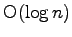adder.

2. Recall:
1. Carry generate: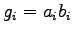.

2. Carry propagate: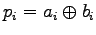.

Restricting the carry computation circuitry to a tree structure: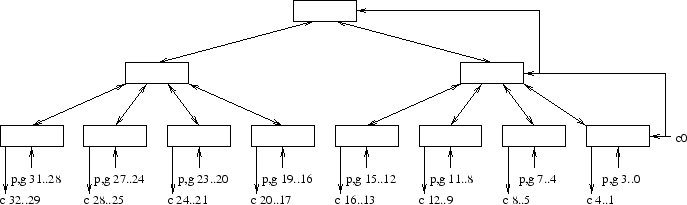• Non-Leaves: Four-bit carry lookahead group units.

Block diagram: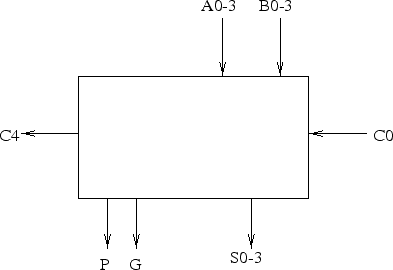Block generate, propagate.

2. What is the fan-in?

3. What is the delay model from inputs to outputs?

1. Design a 4-Group carry lookahead unit.

Block diagram: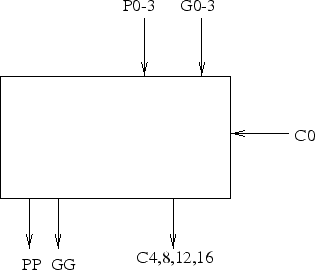Use of block generates, propagates.

2. What is the fan-in?

3. What is the delay model from inputs to outputs?

Total gate delays for ripple-carry adder.

Signed Digit Representations

1. Consider the digit set of the maximally redundant signed digit representation for radix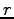: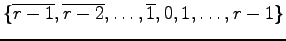2. For radix 2 we have: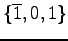.

Radix 4: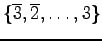.

3. For some values, there are multiple representations. For example: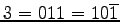(radix 2).

4. This redundancy can be exploited so that we can design constant time signed digit adders.

1. Idea: Ensure that a carry propagates no further than two bit positions.

2. Circuit sketch: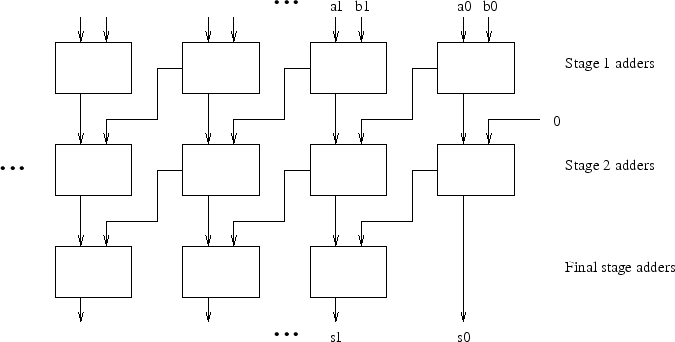Addend + Augend Carry Sum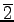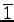01 0 0 0 1 0 1 2 1 0
Goal: Ensure sums are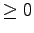to eliminate -2 as a possible starting sum in the next stage.

 Addend + Augend Carry Sum00 0 0 1 12 1 0
Goal: Ensure sums are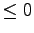to eliminate 2 and -2 as possible starting sums in the next stage.
 Addend + Augend Carry Sum00 0 0 1 0 1
Tom Kelliher